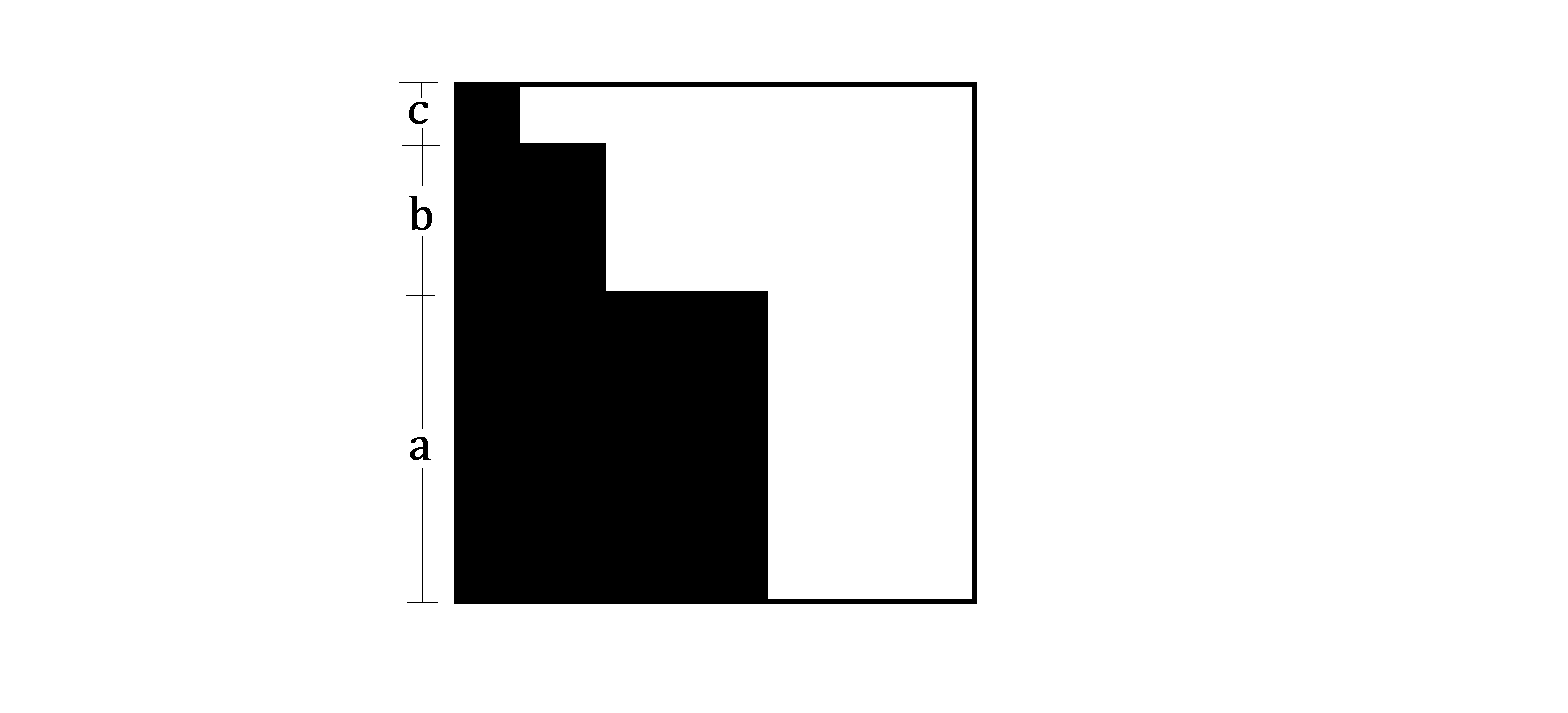# Present Wrap

Geometry Level 2A big square sheet of wrapping paper is cut out as the squares of side lengths $a$, $b$, and $c$, respectively, as shown above.

Will the rest of the paper be enough to wrap a present box of dimensions $a\times b\times c$?

×